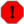## tablei

tablei — Accesses table values by direct indexing with linear interpolation.

## Description

Accesses table values by direct indexing with linear interpolation.

## Syntax

`ares tablei andx, ifn [, ixmode] [, ixoff] [, iwrap]`
`ires tablei indx, ifn [, ixmode] [, ixoff] [, iwrap]`
`kres tablei kndx, ifn [, ixmode] [, ixoff] [, iwrap]`

## Initialization

ifn -- function table number. tablei requires the extended guard point.

ixmode (optional) -- index data mode. The default value is 0.

• 0 = raw index

• 1 = normalized (0 to 1)

ixoff (optional) -- amount by which index is to be offset. For a table with origin at center, use tablesize/2 (raw) or .5 (normalized). The default value is 0.

iwrap (optional) -- wraparound index flag. The default value is 0.

• 0 = nowrap (index < 0 treated as index=0; index > tablesize sticks at index=size)

• 1 = wraparound.

## Performance

tablei is a interpolating unit in which the fractional part of index is used to interpolate between adjacent table entries. The smoothness gained by interpolation is at some small cost in execution time (see also oscili, etc.), but the interpolating and non-interpolating units are otherwise interchangeable. Note that when tablei uses a periodic index whose modulo n is less than the power of 2 table length, the interpolation process requires that there be an (n + 1)th table value that is a repeat of the 1st (see f Statement in score).

WarningReading tables which contain stereo- or multichannel-information probably causes unwanted noise as the opcode interpolates between subsequent positions of the table regardless of the origin of its content. Usually just the content of one channel is expected Consider using loscilx instead. b

## Examples

Here is an example of the tablei opcode. It uses the file tablei.csd.

Example 1069. Example of the tablei opcode.

See the sections Real-time Audio and Command Line Flags for more information on using command line flags.

```<CsoundSynthesizer>
<CsOptions>
; Select audio/midi flags here according to platform
-odac     ;;;realtime audio out
; For Non-realtime ouput leave only the line below:
; -o tablei.wav -W ;;; for file output any platform
</CsOptions>
<CsInstruments>

sr = 44100
ksmps = 32
nchnls = 2
0dbfs  = 1

seed 0	;generate new values every time the instr is played

instr 1

ifn = p4
isize = p5
ithresh = 0.5

itemp ftgen ifn, 0, isize, 21, 2

iwrite_value = 0
i_index = 0

loop_start:

iwrite_value = 1
else
iwrite_value = -1
endif
tableiw iwrite_value, i_index, ifn
loop_lt i_index, 1, isize, loop_start
turnoff

endin

instr 2

ifn = p4
isize = ftlen(ifn)
prints "Index\tValue\n"

i_index = 0
loop_start:
ivalue tablei i_index, ifn
prints "%d:\t%f\n", i_index, ivalue

loop_lt i_index, 1, isize, loop_start		;read table 1 with our index

aout oscili .5, 100, ifn			;use table to play the polypulse
outs   aout, aout

endin
</CsInstruments>
<CsScore>
i 1 0 1 100 16
i 2 0 2 100
e
</CsScore>
</CsoundSynthesizer>
```